# SOCI8015 Lab 5: Univariate Statistics

The fifth lab session covers the following:

• How to make a frequency table
• How to compute the mode
• How to calculate descriptive statistics
• How to visualise the distribution

We will use four packages for this lab. Load them using the following code:

library(sjlabelled)
library(sjmisc)
library(sjPlot)
library(summarytools)

Note that the loading order of packages is essential. If you do not follow the suggested loading order, you will not get the same result as in the all below examples.

Also, you may see a error messages especially when you load summarytools. Please ignore them. It will not affect your analytic outcomes.

# Import the 2009 AuSSa dataset.

This lab uses the 2009 AuSSa dataset. You can download the file of this dataset in the course website(iLearn). Download the data file and put it into your working directory. Then, run the following code:

aus2009 <-readRDS("aussa2009.rds")

The dataset is loaded as aus2009.

# How to make a frquency table

I briefly introduced how to make a frequency table in Lab 4. ‘frq(data name$variable name)’ generates a frequency table. For instance, the following code produces a frequency table of sex in aus2009: frq(aus2009$sex) 
## R: Sex (x) <numeric>
## # total N=1525 valid N=1494 mean=1.57 sd=0.50
##
## Value |  Label |   N | Raw % | Valid % | Cum. %
## -----------------------------------------------
##     1 |   Male | 647 | 42.43 |   43.31 |  43.31
##     2 | Female | 847 | 55.54 |   56.69 | 100.00
##  <NA> |   <NA> |  31 |  2.03 |    <NA> |   <NA>

In the outcome, NA means missing values (item non-responses). frq is frequencies, raw.prc denotes raw percentages (based on the number of total cases), valid.prc indicates valid percentages (based on the number of valid cases), and cum.prc means cumulative percentages.

Alternatively, you can make a frequency table using ‘freq()’ from summarytools package. I prefer to use ‘freq()’ because this function provides more detailed information.

freq(aus2009$sex)  ## Frequencies ## aus2009$sex
## Label: R: Sex
## Type: Numeric
##
##               Freq   % Valid   % Valid Cum.   % Total   % Total Cum.
## ----------- ------ --------- -------------- --------- --------------
##           1    647     43.31          43.31     42.43          42.43
##           2    847     56.69         100.00     55.54          97.97
##        <NA>     31                               2.03         100.00
##       Total   1525    100.00         100.00    100.00         100.00

The problem of this output is that value labels are not shown in the frequency table. This is because summarytools package does not support the value labels assigned by sjlabelled package. To show value labels, we need to add one more function: ‘to_label()’.

freq(to_label(aus2009$sex)) ## Frequencies ## ## Freq % Valid % Valid Cum. % Total % Total Cum. ## ------------ ------ --------- -------------- --------- -------------- ## Male 647 43.31 43.31 42.43 42.43 ## Female 847 56.69 100.00 55.54 97.97 ## <NA> 31 2.03 100.00 ## Total 1525 100.00 100.00 100.00 100.00 Now, you can see the labels of all values in the frequency table. In the table, Freq is frequencies, % Valid indicates valid percentages (based on the number of valid cases), and % Valid Cum. means cumulative percentages based on valid cases, % Total denotes raw percentages (based on the number of total cases), and % Total Cum. is cumulative percentages based on total cases. Also, this frequency table provides the number of total cases at the bottom row. The next codes will create frequency tables of marital and richcol. freq(to_label(aus2009$marital))
## Frequencies
##
##                                                                Freq   % Valid   % Valid Cum.   % Total   % Total Cum.
## ------------------------------------------------------------ ------ --------- -------------- --------- --------------
##                                                      Married    926     62.11          62.11     60.72          60.72
##                                                      Widowed     81      5.43          67.54      5.31          66.03
##                                                     Divorced    129      8.65          76.19      8.46          74.49
##       Separated (married but sep./not living w legal spouse)     30      2.01          78.20      1.97          76.46
##                                        Never married, single    325     21.80         100.00     21.31          97.77
##                                                         <NA>     34                               2.23         100.00
##                                                        Total   1525    100.00         100.00    100.00         100.00
freq(to_label(aus2009$richcol)) ## Frequencies ## ## Freq % Valid % Valid Cum. % Total % Total Cum. ## -------------------------------- ------ --------- -------------- --------- -------------- ## Strongly agree 133 8.91 8.91 8.72 8.72 ## Agree 378 25.34 34.25 24.79 33.51 ## Neither agree nor disagree 219 14.68 48.93 14.36 47.87 ## Disagree 553 37.06 85.99 36.26 84.13 ## Strongly disagree 209 14.01 100.00 13.70 97.84 ## <NA> 33 2.16 100.00 ## Total 1525 100.00 100.00 100.00 100.00 ## How to make a frequency table of age groups The frequency table of continuous variables is not easy to read because there are so many values in them. Consequently, researchers often transform continuous variables into ordinal ones and then create the frequency table. Let’s make the frequency table of respondents’ age. We will first recode age into a variable of age groups (You did this in Lab 4. see Creating a Variable of Age Groups) and then make the frequency table of age groups. aus2009 <- rec(aus2009, age, rec = "min:19 = 1; 20:29 = 2; 30:39 = 3; 40:49 = 4; 50:59 = 5; 60:69 = 6; 70:79 = 7; 80:89 = 8; 90:max = 9", append = TRUE) aus2009$age_r <- set_label(aus2009$age_r, label = "Age Category") aus2009$age_r <- set_labels(aus2009$age_r, labels = c ("10s" = 1, "20s" = 2, "30s" = 3, "40s" = 4, "50s" = 5, "60s" = 6, "70s" = 7, "80s" = 8, "90s" = 9)) freq(to_label(aus2009$age_r))
## Frequencies
##
##               Freq   % Valid   % Valid Cum.   % Total   % Total Cum.
## ----------- ------ --------- -------------- --------- --------------
##         10s     31      2.09           2.09      2.03           2.03
##         20s    134      9.03          11.12      8.79          10.82
##         30s    182     12.26          23.38     11.93          22.75
##         40s    251     16.91          40.30     16.46          39.21
##         50s    338     22.78          63.07     22.16          61.38
##         60s    302     20.35          83.42     19.80          81.18
##         70s    176     11.86          95.28     11.54          92.72
##         80s     67      4.51          99.80      4.39          97.11
##         90s      3      0.20         100.00      0.20          97.31
##        <NA>     41                               2.69         100.00
##       Total   1525    100.00         100.00    100.00         100.00

# How to compute the mode

R does not have a built-in function to compute a mode of a variable. So, I made a function called ‘getmode()’ which lets you calculate the mode. First, run the following code:

getmode <- function(v) {
uniqv <- unique(v)
uniqv[which.max(tabulate(match(v, uniqv)))]
}

You will see getmode in the tab of Environment. This is the custom function you just added. Thus, every time you want to compute the mode, you need to run the above code first. Let’s caculate the mode of sex, martial, richcol, age and age_r. Again, we will use ’to_label() function for nominal and ordinal variables to see value labels instead of values.

getmode(to_label(aus2009$sex)) ##  Female ## Levels: Male Female getmode(to_label(aus2009$marital))
##  Married
## 5 Levels: Married Widowed ... Never married, single
getmode(to_label(aus2009$richcol)) ##  Disagree ## 5 Levels: Strongly agree Agree Neither agree nor disagree ... Strongly disagree getmode(to_label(aus2009$age))
##  62
getmode(to_label(aus2009$age_r)) ##  50s ## Levels: 10s 20s 30s 40s 50s 60s 70s 80s 90s The results show that ‘Female’ is the mode for sex, ‘Married’ for marital, ‘Disagree’ for richcol, 62 for age, and ‘50s’ for age_r. # How to compute descriptive statistics descr()’ from summarytools package shows various descriptive statistics. The notable statistics include: 1. Mean 2. Std.Dev. (Standard Deviation) 3. Min (Minimum Value) 4. Q1 (25th percentile) 5. Median (50th percentile) 6. Q3 (75th percentile) 7. Max (Maximum Value) 8. IQR (Inter-quartile Range) 9. Skewness 10. N.Valid (Number of Valid Cases) 11. % Valid (Percentage of Valid Cases) The following code shows descriptive statistics of richcol(ordinal variable): descr(aus2009$richcol)
## Descriptive Statistics
## aus2009$richcol ## Label: Q2c Getting ahead: In [Rs country] only the rich can afford the costs of attending university. ## N: 1525 ## ## richcol ## ----------------- --------- ## Mean 3.22 ## Std.Dev 1.22 ## Min 1.00 ## Q1 2.00 ## Median 4.00 ## Q3 4.00 ## Max 5.00 ## MAD 1.48 ## IQR 2.00 ## CV 0.38 ## Skewness -0.26 ## SE.Skewness 0.06 ## Kurtosis -1.09 ## N.Valid 1492.00 ## Pct.Valid 97.84 Please note that in case of ordinal variables, you need to be careful in interpreting the results. For instance, the median value of richcol is 4, which means the median response is “Disagree”. The following code will show descriptive statistics of age(continuous variable): descr(aus2009$age)
## Descriptive Statistics
## aus2009$age ## N: 1525 ## ## age ## ----------------- --------- ## Mean 52.53 ## Std.Dev 16.78 ## Min 17.00 ## Q1 41.00 ## Median 54.00 ## Q3 64.00 ## Max 93.00 ## MAD 17.79 ## IQR 23.00 ## CV 0.32 ## Skewness -0.18 ## SE.Skewness 0.06 ## Kurtosis -0.68 ## N.Valid 1484.00 ## Pct.Valid 97.31 # How to visualise the distribution There are many packages to visualise your data in R. In this unit I will use sjPlot package because it supports the labels assigned by sjlabelled package and is easy to use. If you want to learn more advanced graphics of R, I recommend using ggplot2 package. One good reliable online resource is here. Note: The graph or chart will be displayed in the tab of Plots at the bottom left pane. Sometimes the window of Plots is too small to show the whole graph. Thus, you may need to change the size of the Plots window. To change the size of the Plots window (see <Figure 1>), 1. Move the cursor at the border between top left and bottom left pane. The icon of mouse cursor will be changed. 2. Hold clicking the mouse and change the height. 3. Move the cursor at the border between left and right panes. The icon of mouse cursor will be changed. 4. Hold clicking the mouse and change the width.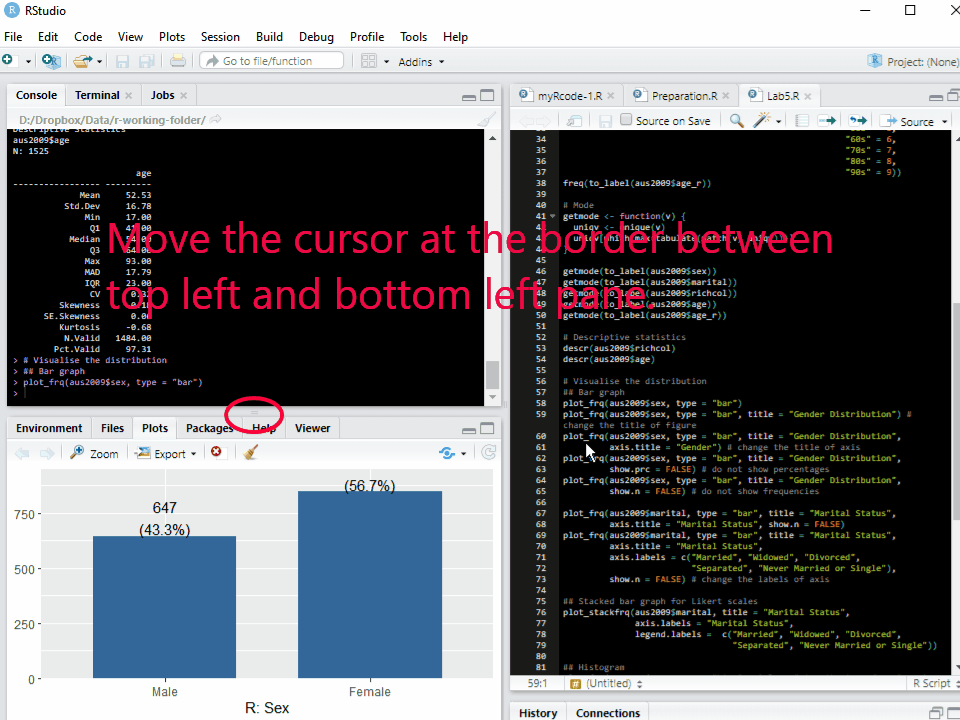Figure 1: Changing the Size of Plots Window ## How to make bar graphs Bar graphs provide appropriate ways to present nominal or ordinal variables graphically. ‘plot_frq(data name$variable name, type = "bar")’ makes a bar graph of the specified variable. For example, the following code will make a bar graph of sex in aus2009:

plot_frq(aus2009$sex, type = "bar")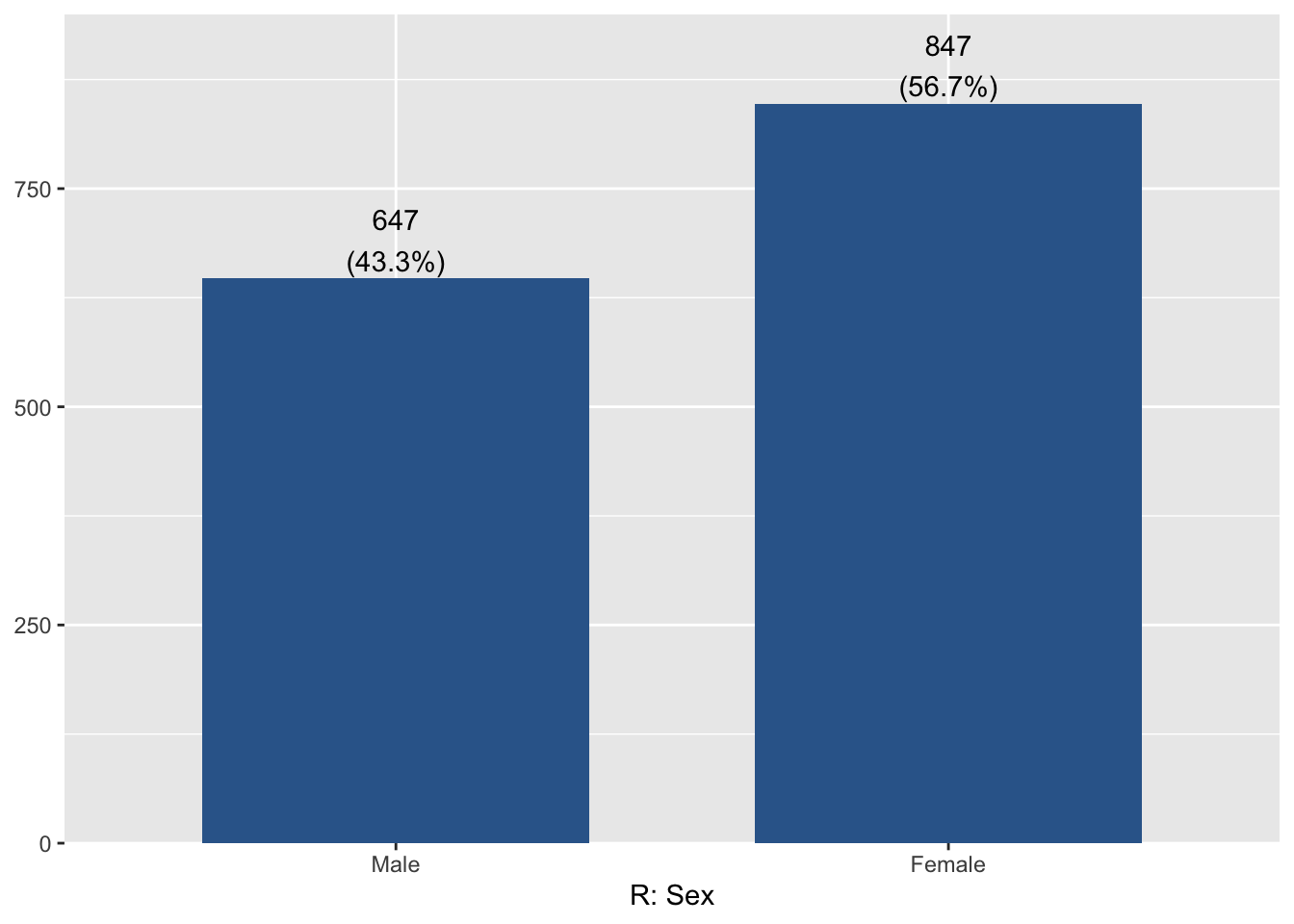If you want to add the title of a figure, add ‘title = "Any title"’ to the code. The following code will add “Gender distribution” as the title of the figure: plot_frq(aus2009$sex, type = "bar", title = "Gender Distribution")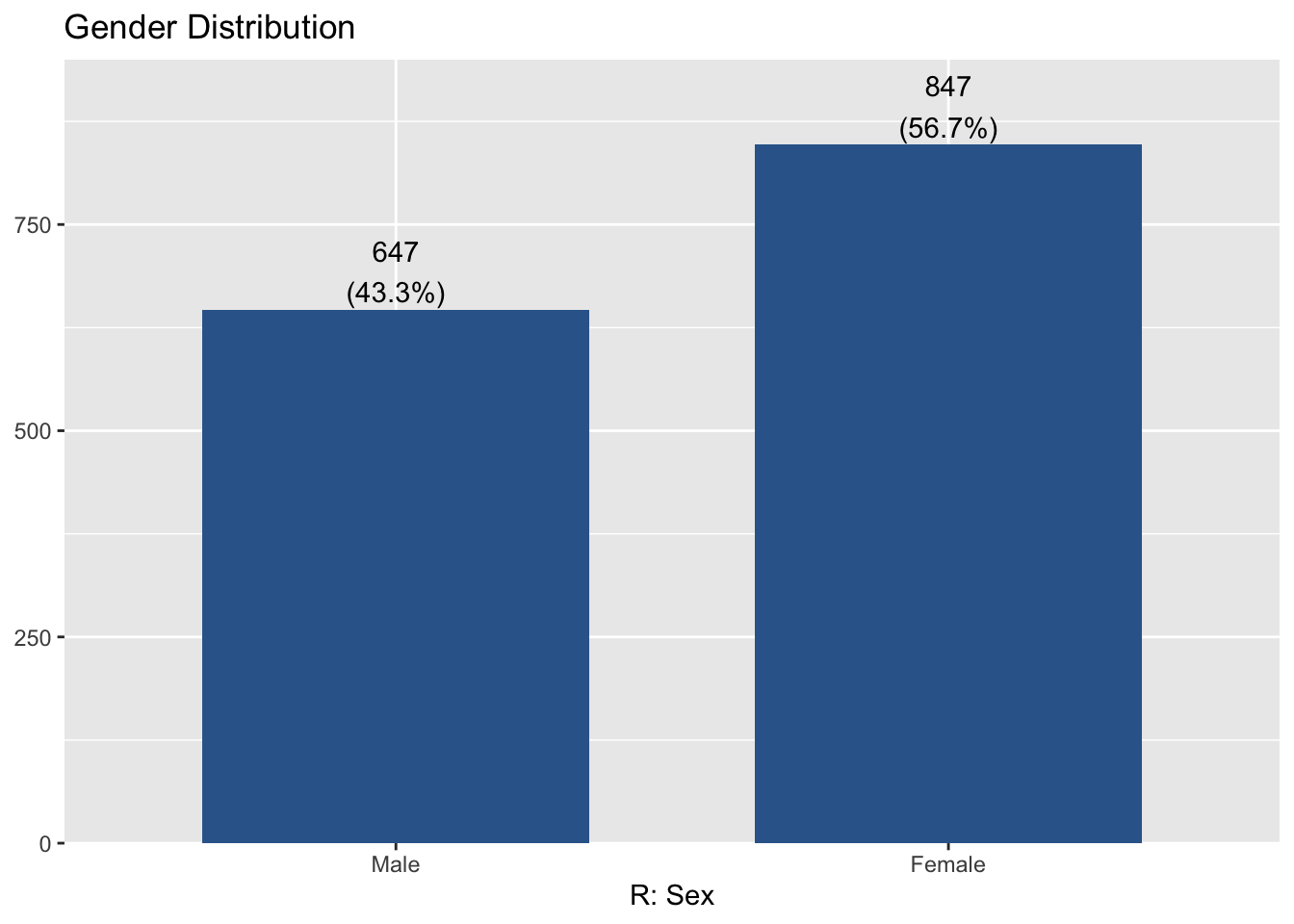The title of X-axis(R: Sex) is somewhat bizarre. The title of X-axis can be changed by adding ‘axis.title = "Any title"’. I change it into “Gender”. Thus, the code is:

plot_frq(aus2009$sex, type = "bar", title = "Gender Distribution", axis.title = "Gender")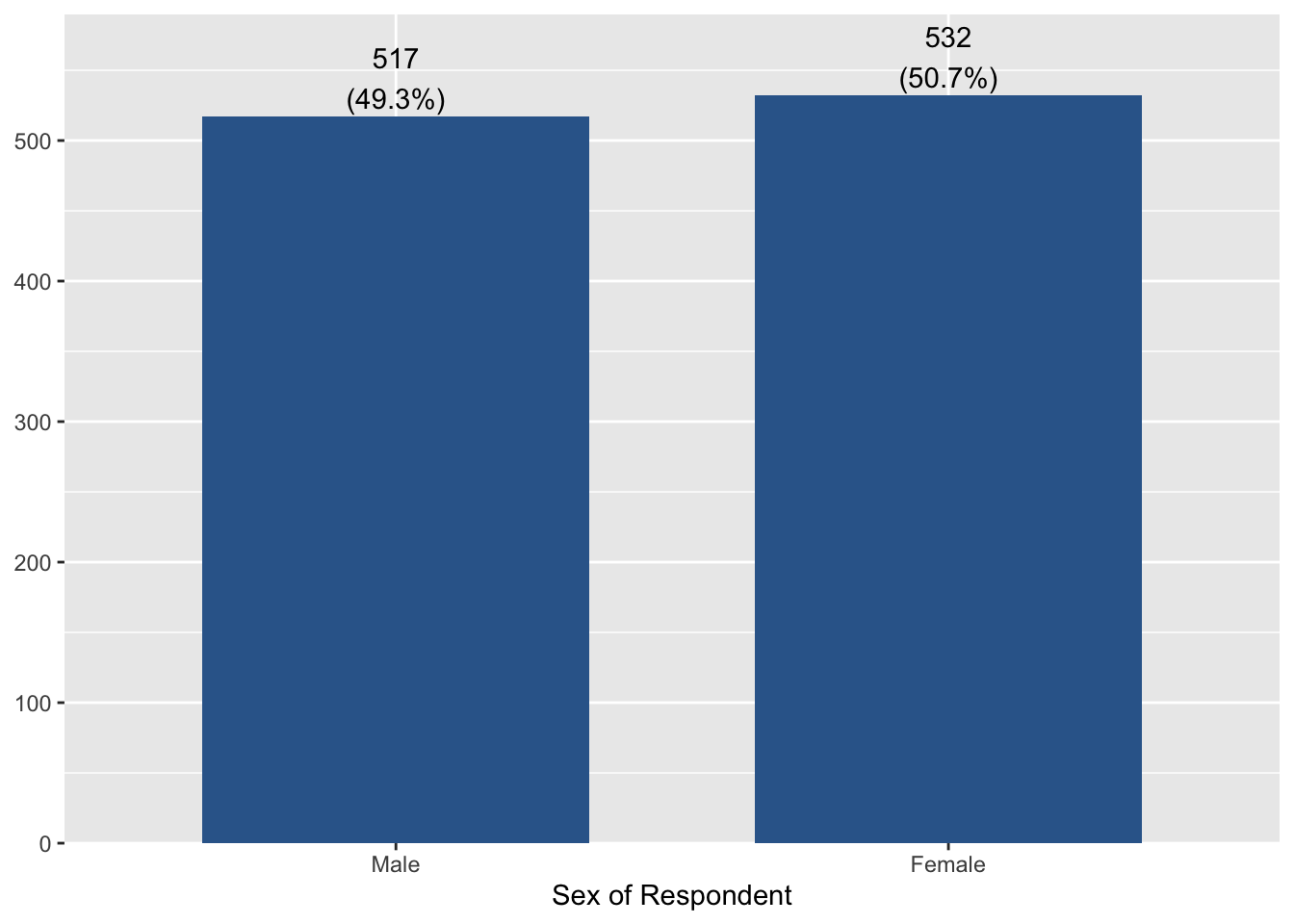If you want to remove percentages of each category at the top of bars, add ‘show.prc = FALSE’ to the code. plot_frq(aus2009$sex, type = "bar", title = "Gender Distribution",
show.prc = FALSE)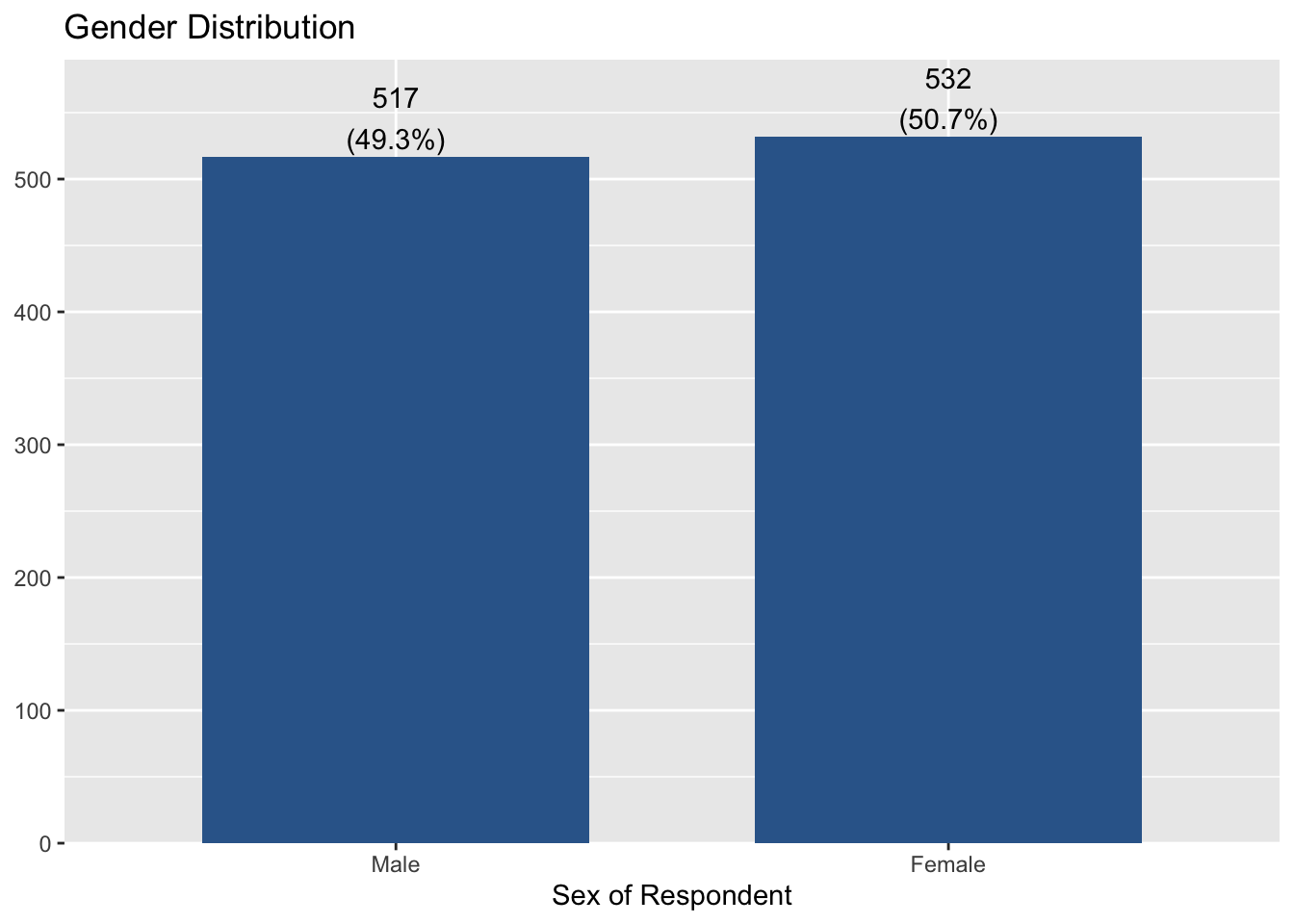If you want to remove frequencies of each category at the top of bars, add ‘show.n = FALSE’ to the code.

plot_frq(aus2009$sex, type = "bar", title = "Gender Distribution", show.n = FALSE)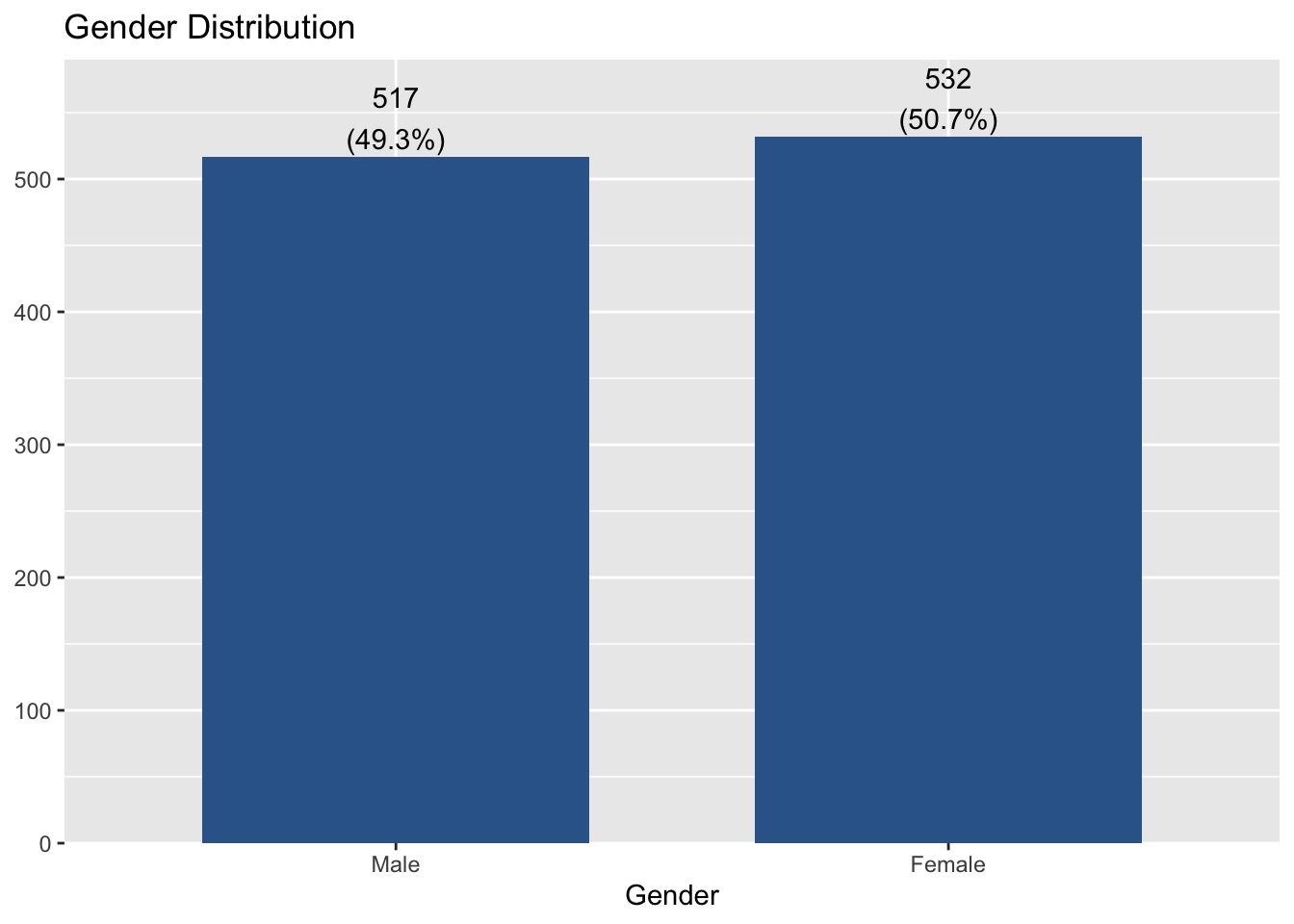Next, let’s make a bar graph of another variable, marital. plot_frq(aus2009$marital, type = "bar", title = "Marital Status",
axis.title = "Marital Status", show.n = FALSE)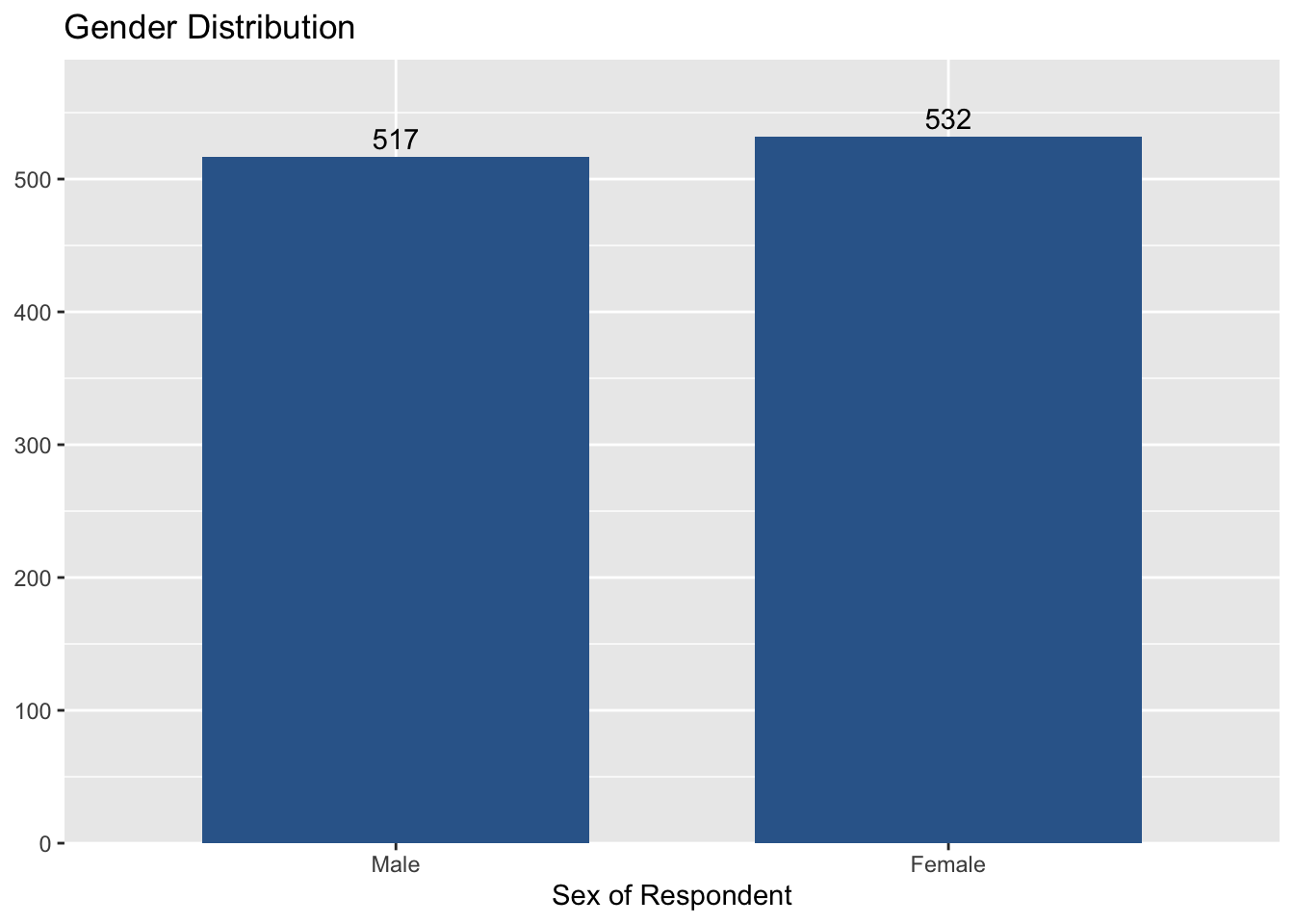You may notice that one axis label is too long, which makes it difficult to read other labels. So, we will change this long label into a more brief one. To change the axis label, ‘axis.labels’ option will be used.

plot_stackfrq(aus2009$marital, title = "Marital Status", axis.labels = "Marital Status", legend.labels = c("Married", "Widowed", "Divorced", "Separated", "Never Married or Single"))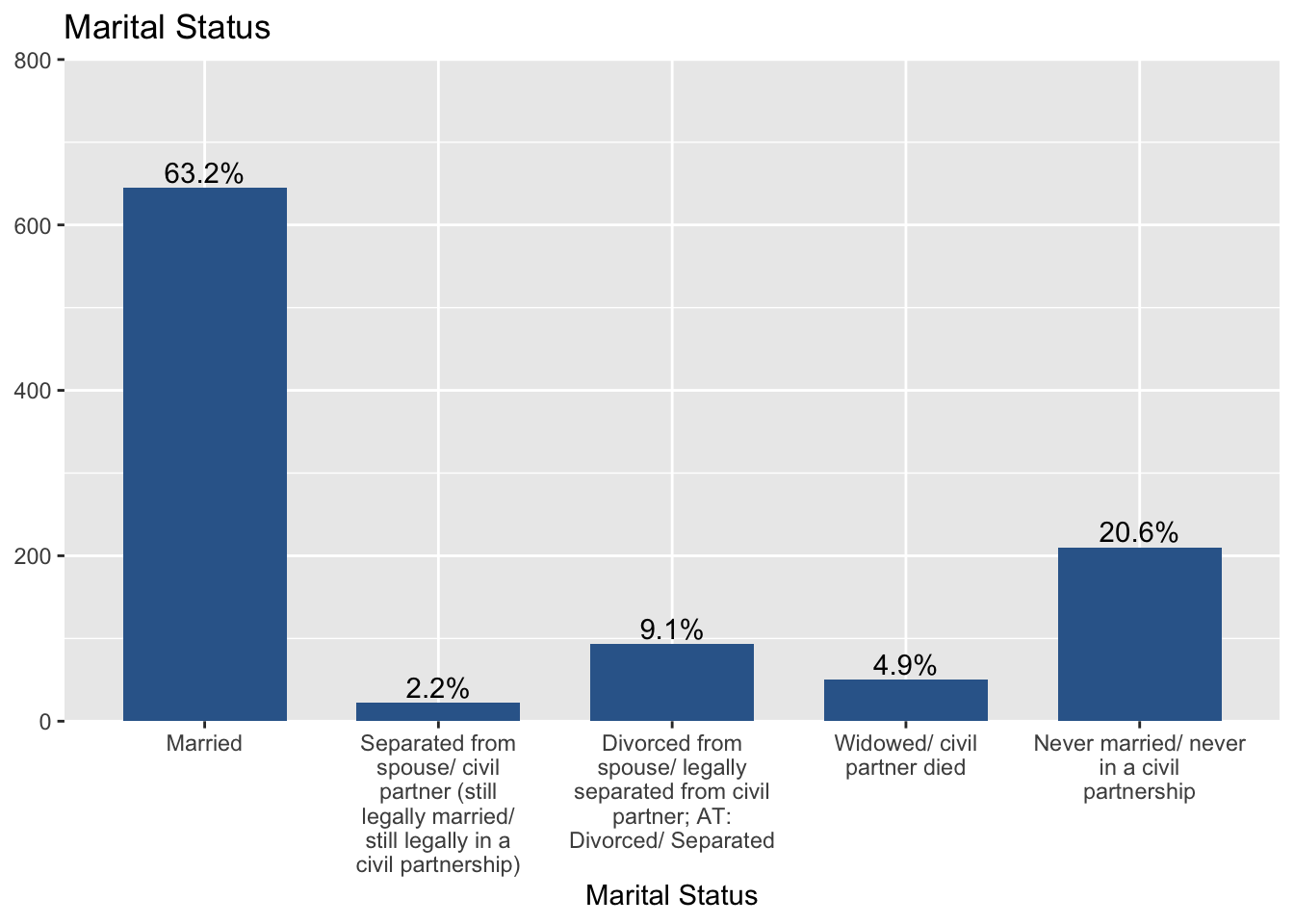Note that I changed the label of axis and legend using ‘axis.labels’ and ‘legend.labels’ option. ## How to make histograms Histograms are used for continuous variables which have many scores. Histograms look like bar graphs, except that the sides of the “bars” touch to form a continuous series. To make histograms, use ‘plot_frq(data name$variable name, type = "hist")’. The following code creates a histogram of age.

plot_frq(aus2009$age, type = "hist", title = "Distribution of Age", axis.title = "Age")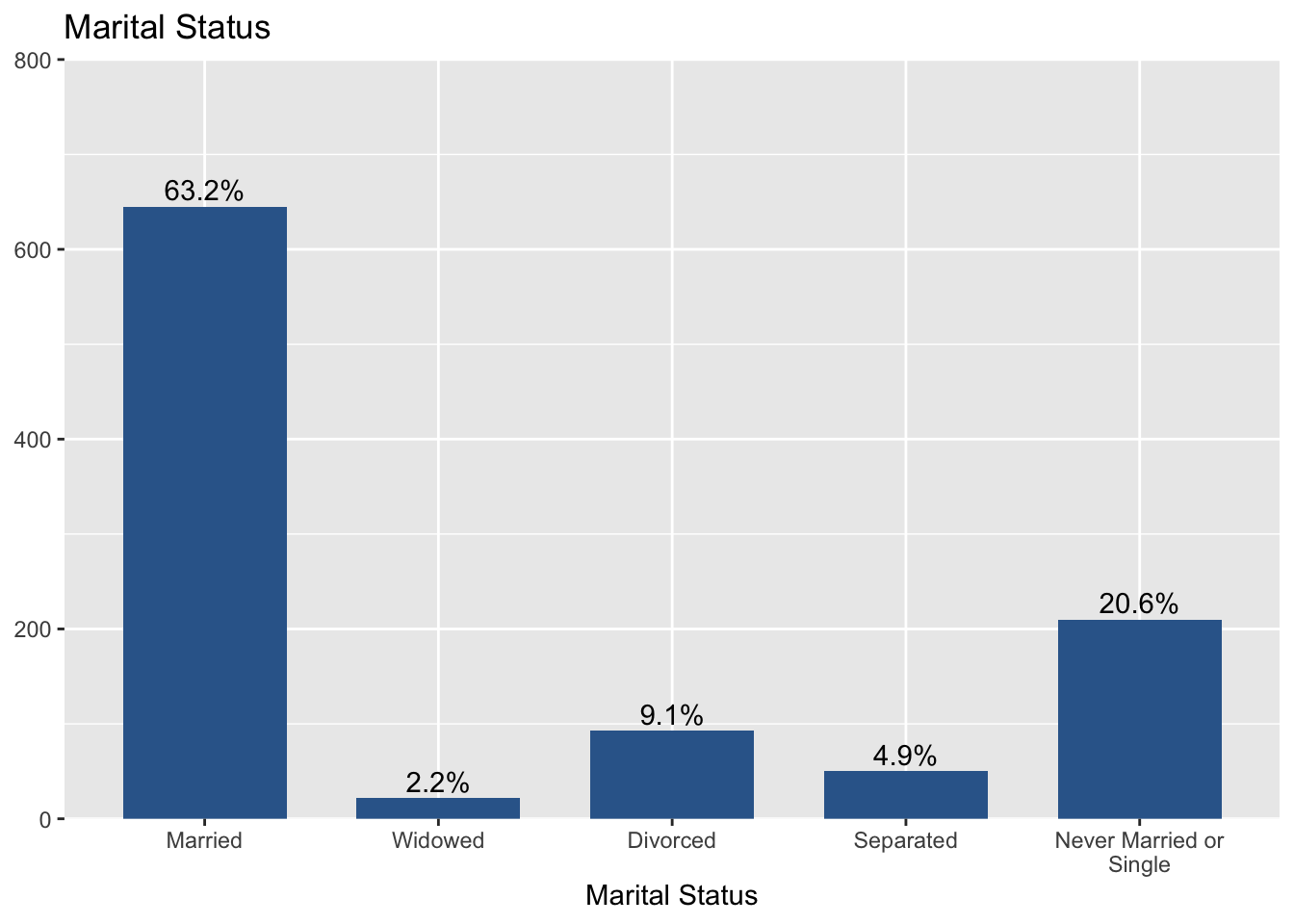In this graph, I would like to set the distance between axis tick labels equal to 10. So, I add ‘grid.breaks = 10’ to the code. Thus, the code is: plot_frq(aus2009$age, type = "hist", title = "Distribution of Age", axis.title = "Age",
grid.breaks = 10)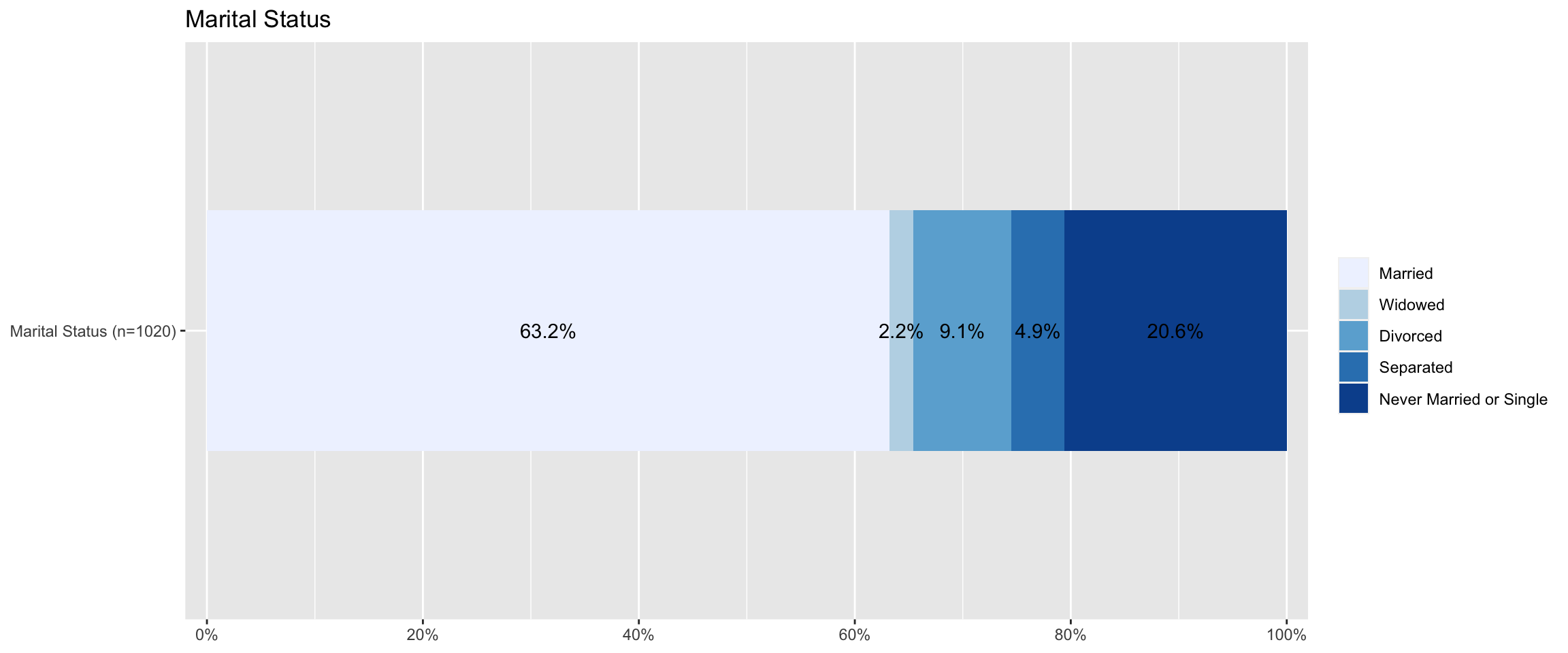# Save the dataset

Save your dataset. Otherwise, you will lose a newly constructed variable (age_r).

saveRDS(aus2009, file = "aussa2009.rds")

# Lab 5 Participation Activity

1. Did you successfully make the frequency tables of the following four variables: sex, marital, richcol and age_r?

1. Did you successfully compute the mode of the following five variables: sex, marital, richcol, age and age_r?

1. Did you successfully compute the descriptive statistics of richcol and age?

1. Did you successfully make bar graphs, stacked bar graphs and histograms as instructed?

1. After completing all the tasks in this lab, did you successfully save the data in the format of RDS?

Note: Please complete the Lab 5 Participation Activity. You can find the link to this activity on iLearn. This activity will contribute to your participation marks.

The R codes you have written so far look like:

################################################################################
# Lab 5: Univariate Analysis
# 28/03/2022
# SOCI8015 & SOCX8015
################################################################################

library(sjlabelled)
library(sjmisc)
library(sjPlot)
library(summarytools)

# Frequency table
frq(aus2009$sex) freq(aus2009$sex)
freq(to_label(aus2009$sex)) freq(to_label(aus2009$marital))
freq(to_label(aus2009$richcol)) # Make a frequency table of age gropus aus2009 <- rec(aus2009, age, rec = "min:19 = 1; 20:29 = 2; 30:39 = 3; 40:49 = 4; 50:59 = 5; 60:69 = 6; 70:79 = 7; 80:89 = 8; 90:max = 9", append = TRUE) aus2009$age_r <- set_label(aus2009$age_r, label = "Age Category") aus2009$age_r <- set_labels(aus2009$age_r, labels = c ("10s" = 1, "20s" = 2, "30s" = 3, "40s" = 4, "50s" = 5, "60s" = 6, "70s" = 7, "80s" = 8, "90s" = 9)) freq(to_label(aus2009$age_r))

# Mode
getmode <- function(v) {
uniqv <- unique(v)
uniqv[which.max(tabulate(match(v, uniqv)))]
}

getmode(to_label(aus2009$sex)) getmode(to_label(aus2009$marital))
getmode(to_label(aus2009$richcol)) getmode(to_label(aus2009$age))
getmode(to_label(aus2009$age_r)) # Descriptive statistics descr(aus2009$richcol)
descr(aus2009$age) # Visualise the distribution ## Bar graph plot_frq(aus2009$sex, type = "bar")
plot_frq(aus2009$sex, type = "bar", title = "Gender Distribution") # change the title of figure plot_frq(aus2009$sex, type = "bar", title = "Gender Distribution",
axis.title = "Gender") # change the title of axis
plot_frq(aus2009$sex, type = "bar", title = "Gender Distribution", show.prc = FALSE) # do not show percentages plot_frq(aus2009$sex, type = "bar", title = "Gender Distribution",
show.n = FALSE) # do not show frequencies

plot_frq(aus2009$marital, type = "bar", title = "Marital Status", axis.title = "Marital Status", show.n = FALSE) plot_frq(aus2009$marital, type = "bar", title = "Marital Status",
axis.title = "Marital Status",
axis.labels = c("Married", "Widowed", "Divorced",
"Separated", "Never Married or Single"),
show.n = FALSE) # change the labels of axis

## Stacked bar graph for Likert scales
plot_stackfrq(aus2009$marital, title = "Marital Status", axis.labels = "Marital Status", legend.labels = c("Married", "Widowed", "Divorced", "Separated", "Never Married or Single")) ## Histogram plot_frq(aus2009$age, type = "hist", title = "Distribution of Age", axis.title = "Age")
plot_frq(aus2009\$age, type = "hist", title = "Distribution of Age", axis.title = "Age",
grid.breaks = 10) # grid.breks:sets the distance between breaks for the axis

# Save dataset
saveRDS(aus2009, file = "aussa2009.rds")
Last updated on 24 March, 2022 by Dr Hang Young Lee(hangyoung.lee@mq.edu.au)